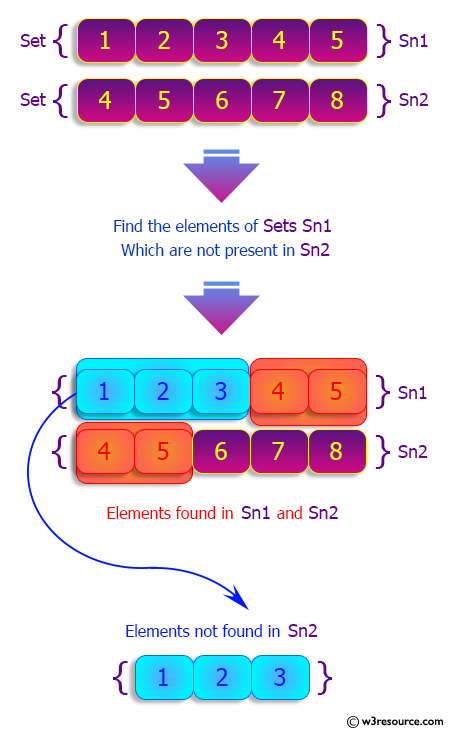﻿ Python: Find the elements in a given set that are not in another set - w3resource# Python: Find the elements in a given set that are not in another set

## Python sets: Exercise-19 with Solution

Write a Python program to find the elements in a given set that are not in another set.

Sample Solution:

Python Code:

``````sn1 = {1,2,3,4,5}
sn2 = {4,5,6,7,8}
print("Original sets:")
print(sn1)
print(sn2)
print("Difference of sn1 and sn2 using difference():")
print(sn1.difference(sn2))
print("Difference of sn2 and sn1 using difference():")
print(sn2.difference(sn1))
print("Difference of sn1 and sn2 using - operator:")
print(sn1-sn2)
print("Difference of sn2 and sn1 using - operator:")
print(sn2-sn1)
```
```

Sample Output:

```Original sets:
{1, 2, 3, 4, 5}
{4, 5, 6, 7, 8}
Difference of sn1 and sn2 using difference():
{1, 2, 3}
Difference of sn2 and sn1 using difference():
{8, 6, 7}
Difference of sn1 and sn2 using - operator:
{1, 2, 3}
Difference of sn2 and sn1 using - operator:
{8, 6, 7}
```

Pictorial Presentation:## Visualize Python code execution:

The following tool visualize what the computer is doing step-by-step as it executes the said program:

Python Code Editor:

Have another way to solve this solution? Contribute your code (and comments) through Disqus.

What is the difficulty level of this exercise?

Test your Python skills with w3resource's quiz

﻿

## Python: Tips of the Day

While-else construct:

```i = 5

while i > 1:
print("Whil-ing away!")
i -= 1
if i == 3:
break
else:
print("Finished up!")
```